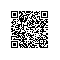# 排序高级之交换排序_快速排序

1. 从数列中挑出一个元素，称为 "基准"（pivot），
2. 重新排序数列，所有元素比基准值小的摆放在基准前面，所有元素比基准值大的摆在基准的后面（相同的数可以到任一边）。在这个分区退出之后，该基准就处于数列的中间位置。这个称为分区（partition）操作。
3. 递归地（recursive）把小于基准值元素的子数列和大于基准值元素的子数列排序。

## 正规的分析

T(n) = O(n) + 2T(n/2)

T(n) = O(n) + T(1) + T(n - 1) = O(n) + T(n - 1)

### 平均复杂度$C(n) = n - 1 + \frac{1}{n} \sum_{i=0}^{n-1} (C(i)+C(n-i-1)) = 2n \ln n = 1.39n \log_2 n.$

### 空间复杂度$\sum_{i=0}^{\infty} \frac{n}{2^i} = 2n.$$\sum_{i=0}^n (n-i+1) = \Theta (n^2)$

最差时间复杂度$\Theta(n^2)$$\Theta(n\log n)$$\Theta(n\log n)$ 根据实现的方式不同而不同1. package com.baobaotao.test;
2. /**
3.  * 排序研究
4.  * @author benjamin(吴海旭)
5.  * @email benjaminwhx@sina.com / 449261417@qq.com
6.  *
7.  */
8. public class Sort {
9.
10.     /**
11.      * 快速排序实现
12.      * @param array
13.      * @param low
14.      * @param high
15.      */
16.     public static void quickSort(int[] array, int low, int high) {
17.         if(low < high) {
18.             int pivot = partition(array, low, high);
19.
20.             quickSort(array, low, pivot - 1);
21.
22.             quickSort(array, pivot + 1, high);
23.         }
24.     }
25.
26.     /**
27.      * 根据传入的最低位和最高位分割数组
28.      * @param array 待排序数组
29.      * @param low   数组下标下界
30.      * @param high  数组上标上界
31.      * @return pivot
32.      */
33.     public static int partition(int[] array, int low, int high) {
34.         //第一个元素所在位置
35.         int p_pos = low ;
36.         //采用第一个元素为轴
37.         int pivot = array[p_pos] ;
38.         for (int i = low + 1; i <= high; i++) {
39.             if (array[i] < pivot) {
40.                 p_pos++;
41.                 swap(array, p_pos, i);
42.             }
43.
44.         }
45.         swap(array, low, p_pos);
46.
47.         return p_pos;
48.     }
49.
50.     /**
51.      * 按从小到大的顺序交换数组
52.      * @param a 传入的数组
53.      * @param b 传入的要交换的数b
54.      * @param c 传入的要交换的数c
55.      */
56.     public static void swap(int[] a, int b, int c) {
57.         if(b == c) return ;
58.         int temp = a[b] ;
59.         a[b] = a[c] ;
60.         a[c] = temp ;
61.     }
62.
63.     /**
64.      * 打印数组
65.      * @param array
66.      */
67.     public static void printArr(int[] array) {
68.         for(int c : array) {
69.             System.out.print(c + " ");
70.         }
71.         System.out.println();
72.     }
73.
74.     public static void main(String[] args) {
75.         int[] number={11,95,45,15,78,84,51,24,12} ;
76.         quickSort(number, 0, number.length-1) ;
77.         printArr(number) ;
78.     }
79. }使用钉钉扫一扫加入圈子
+ 订阅